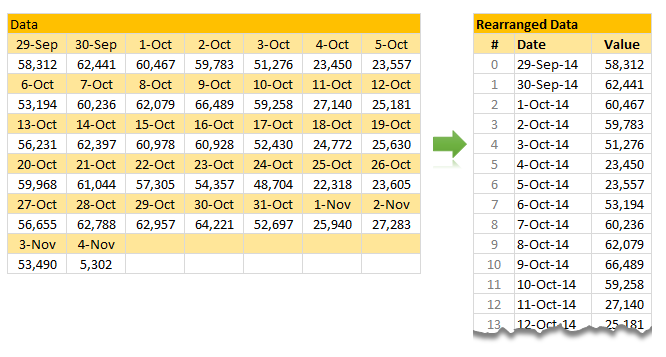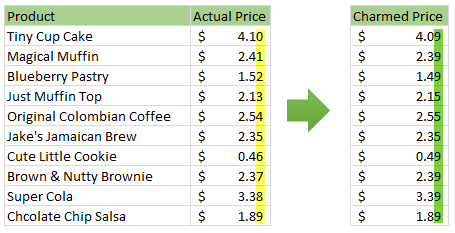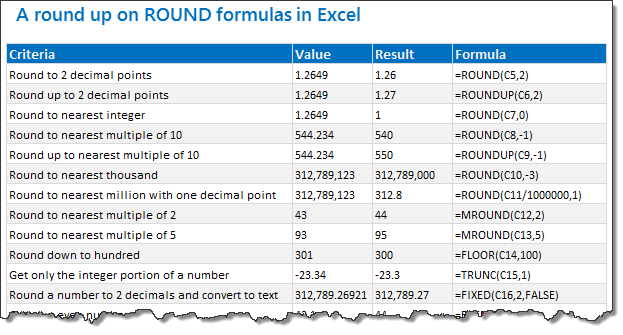# All articles with 'INT()' Tag

## Figure out slot from given time [quick tip]

Published on Apr 19, 2016 in Excel Howtos, Quick TipHere is an interesting scenario.

Let’s say you are looking at a time, like 9:42 AM and want to know which 15 minute slot it fits into. The answer is 9:30 – 9:45. But how would you get this answer thru Excel formulas?

Continue »

## Looking up when data won’t play nice – few more alternatives

Published on Nov 12, 2014 in Learn Excel, VBA MacrosRecently, we discussed about the case of unwieldy data and how we lookup what we want using formulas like SUMIFS. Today, let us learn few more ways to solve the same problem.

Suitable structure spawns simple solutions

Poorly structured is the 2nd biggest problem of analysts. The first one is not enough coffee. That is why there is a dictum in the data analytics world.

Structure is everything

So, we can easily solve our lookup problem, if our data were to magically re-arranged in 2 column fashion – Data & Value.

Continue »

## Charmed Price Problem

Published on Oct 6, 2014 in Excel HowtosHere is a charming little problem to kick start your day.

Lets say you run a cute little bakery around the corner. Since you want your prices to look charming, you have a policy to round them down or up based on below rule.

If the price ends with 0, 1 or 2 cents, round it down to 9 cents.

If the price ends with 3, 4 or 5 cents, round it up to 5 cents.

If the price ends with 6, 7, 8 or 9 cents, round it up to 9 cents.

For example,

So how do you round to nearest charmed price? You could do it manually. But you would rather bake a few more of those Tiny Cup Cakes than waste time rounding the prices. So you want an automatic way to round prices. This is where Excel helps.

Continue »

## 18.2 Tips on Rounding numbers using Excel Formulas

Published on Sep 28, 2012 in Excel Howtos, Learn ExcelLets talk round numbers today.I have 18.2 tips for you on round numbers.

We can use a variety of formulas to round numbers in Excel depending on the situation. We have ROUND, ROUNDUP, ROUNDDOWN, MROUND, INT, TRUNC, CEILING, FLOOR, FIXED, EVEN, ODD and few more. To know how to use all these formulas and how to round numbers based on any criteria, just read on.

Continue »

## Formula Forensics 024. Is this number a Prime Number ?

Published on Jul 12, 2012 in Formula Forensics, Huis, Posts by HuiIs my number a Prime Number?
What is a Prime Number?

Today in Formula Forensics we answer both questions.

Continue »

## Splitting a number into integer and decimal portions

Published on Nov 22, 2010 in Excel Howtos, Learn ExcelHere is a quick formula tip to start another awesome week.

Often while working with data, I need to split a number in to integer and decimal portions. Now, there are probably a ton of ways you can do this. But here are two formulas I use quite often and they work well.

Assuming the number is in cell A1,

• Integer part =INT(A1)
• Decimal part =MOD(A1,1)

These formulas work whenever my data has only positive numbers (which is the case 90% 0f time). But if I am dealing with a mix of positive and negative numbers, …

Continue »

## Array formula to check if a number is prime [just for fun]

Published on May 29, 2009 in Learn Excel

I am math-geek-wannabe, if there ever is such a category. During my 3rd year of graduation I went and purchased the volume 2 of Donald Knuth’s Art of Computer Programming and thus began my love with all things random and prime. I never really became the math-geek I always wanted to, instead I became an insurance expert with tons of passion for data and visualization. But when I get a chance to poke with randomness or numbers, I always lap it up with joy. And that brings us to an interesting array formula trick to check if a number is prime or not.

(assuming the number is in the cell B2) type the below formula and

=IF(MIN(MOD(\$B\$5,ROW(INDIRECT(“2:”&INT(SQRT(\$B\$5))))))=0,”not prime”,”prime”)

hit ctrl+shift+enter and bingo, it tells you if the number is prime or not. Read on to find how it works

Continue »

### Get FREE Excel & Power-BI Newsletter

One email per week with Excel and Power BI goodness. Join 100,000+ others and get it free.